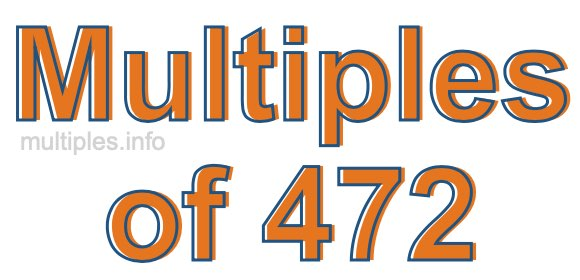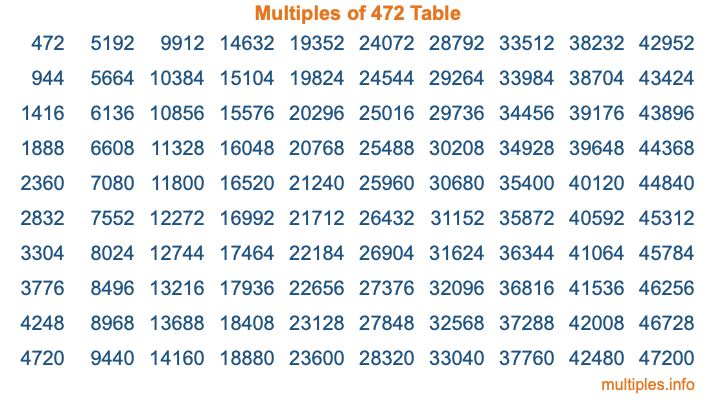Multiples of 472Welcome to the Multiples of 472 page. Here we will first teach you everything you will ever need to know about the multiples of 472, and then give you a study guide summary of everything we taught you to make sure you remember it all. Use this page to look up facts and learn information about the multiples of 472. This page will make you a multiples of four hundred seventy-two expert!

Definition of Multiples of 472
Multiples of 472 are all the numbers that when divided by 472 equal an integer. Each of the multiples of 472 are called a multiple. A multiple of 472 is created by multiplying 472 by an integer.

Therefore, to create a list of multiples of 472, you start with 1 multiplied by 472, then 2 multiplied by 472, then 3 multiplied by 472, and so on for as long as you want. Thus, the list of the first five multiples of 472 is 472, 944, 1416, 1888, and 2360. To see a larger list of multiples of 472, see the printable image of Multiples of 472 further down on this page. We also have a category where you can choose any nth multiple of 472.

Multiples of 472 Checker
The Multiples of 472 Checker below checks to see if any number of your choice is a multiple of 472. In other words, it checks to see if there is any number (integer) that when multiplied by 472 will equal your number. To do that, we divide your number by 472. If the the quotient is an integer, then your number is a multiple of 472.

Is  a multiple of 472?

Least Common Multiple of 472 and ...
A Least Common Multiple (LCM) is the lowest multiple that two or more numbers have in common. This is also called the smallest common multiple or lowest common multiple and is useful to know when you are adding our subtracting fractions. Enter one or more numbers below (472 is already entered) to find the LCM.

Check out our LCM Calculator if you need more details about the Least Common Multiple or if you need the LCM for different numbers for adding and subtraction fractions.

nth Multiple of 472
As we stated above, 472 is the first multiple of 472, 944 is the second multiple of 472, 1416 is the third multiple of 472, and so on. Enter a number below to find the nth multiple of 472.

th multiple of 472

Multiples of 472 vs Factors of 472
472 is a multiple of 472 and a factor of 472, but that is where the similarities end. All postive multiples of 472 are 472 or greater than 472. All positive factors of 472 are 472 or less than 472.

Below is the beginning list of multiples of 472 and the factors of 472 so you can compare:

Multiples of 472: 472, 944, 1416, 1888, 2360, etc.

Factors of 472: 1, 2, 4, 8, 59, 118, 236, 472

As you can see, the multiples of 472 are all the numbers that you can divide by 472 to get a whole number. The factors of 472, on the other hand, are all the whole numbers that you can multiply by another whole number to get 472.

It's also interesting to note that if a number (x) is a factor of 472, then 472 will also be a multiple of that number (x).

Multiples of 472 vs Divisors of 472
The divisors of 472 are all the integers that 472 can be divided by evenly. Below is a list of the divisors of 472.

Divisors of 472: 1, 2, 4, 8, 59, 118, 236, 472

The interesting thing to note here is that if you take any multiple of 472 and divide it by a divisor of 472, you will see that the quotient is an integer.

Multiples of 472 Table
Below is an image of the first 100 multiples of 472 in a table. The table is in chronological order, column by column. The first column has the first ten multiples of 472, the second column has the next ten multiples of 472, and so on.The Multiples of 472 Table is also referred to as the 472 Times Table or Times Table of 472. You are welcome to print out our table for your studies.

Negative Multiples of 472
Although not often discussed or needed in math, it is worth mentioning that you can make a list of negative multiples of 472 by multiplying 472 by -1, then by -2, then by -3, and so on, to get the following list of negative multiples of 472:

-472, -944, -1416, -1888, -2360, etc.

Multiples of 472 Summary
Below is a summary of important Multiples of 472 facts that we have discussed on this page. To retain the knowledge on this page, we recommend that you read through the summary and explain to yourself or a study partner why they hold true.

There are an infinite number of multiples of 472.

A multiple of 472 divided by 472 will equal a whole number.

472 divided by a factor of 472 equals a divisor of 472.

The nth multiple of 472 is n times 472.

The largest factor of 472 is equal to the first positive multiple of 472.

472 is a multiple of every factor of 472.

472 is a multiple of 472.

A multiple of 472 divided by a divisor of 472 equals an integer.

472 divided by a divisor of 472 equals a factor of 472.

Any integer times 472 will equal a multiple of 472.

Multiples of a Number
Here you can get the multiples of another number, all with the same attention to detail as we did for multiples of 472 on this page.

Multiples of
Multiples of 473
Did you find our page about multiples of four hundred seventy-two educational? Do you want more knowledge? Check out the multiples of the next number on our list!